# Construction Of Quadrilaterals Solution of TS & AP Board Class 8 Mathematics

#### Construction Of Quadrilaterals Solution of  TS & AP Board Class 8 Mathematics

###### Exercise 3.1

Question 1.

Construct the quadrilaterals with the given measurements. And write steps of construction.

Quadrilateral ABCD with AB = 5.5 cm, BC = 3.5 cm, CD = 4 cm, AD = 5 cm and ∠A = 45o.

GIVEN : In quadrilateral ABCD ,

AB = 5.5 cm

BC = 3.5 cm

CD = 4 cm

∠A = 45o

PROCEDURE :

Step 1 : Draw a rough sketch of the required quadrilateral and mark the given measurements.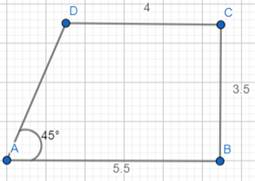Step 2 : Draw ΔDAB using S.A.S property of construction , by taking AD = 5 cm , ∠ DAB = 45° and AB = 5.5 cm.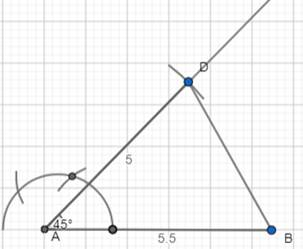Step 3 : To locate the fourth vertex ‘C’ , draw an arc , with center D and radius 4cm (CD=4cm).

Draw another arc with center B and radius 3.5 cm (BC=3.5cm) which cuts the previous arc at C.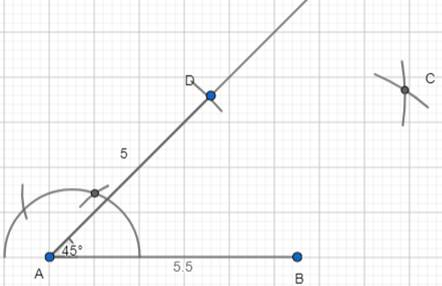Step 4: Join DC and BC to complete the required quadrilateral ABCD.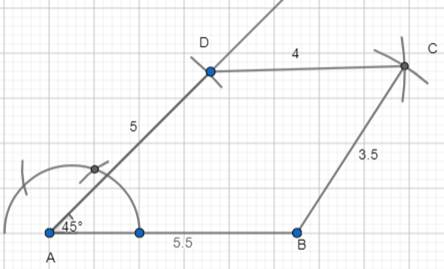Question 2.

Construct the quadrilaterals with the given measurements. And write steps of construction.

Quadrilateral BEST with BE = 2.9 cm, ES = 3.2 cm, ST = 2.7 cm, BT = 3.4 cm and ∠B = 75o.

GIVEN : In quadrilateral ABCD ,

BE = 2.9 cm

ES = 3.2 cm

ST = 2.7 cm

BT = 3.4 cm

∠B = 45o

PROCEDURE :

Step 1 : Draw a rough sketch of the required quadrilateral and mark the given measurements.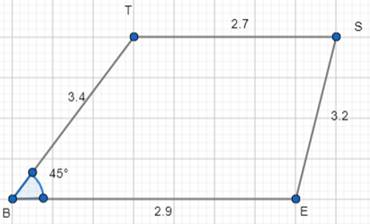Step 2 : Draw ΔTBE using S.A.S property of construction , by taking BT = 3.4 cm , ∠ TBE = 75° and BE = 2.9 cm.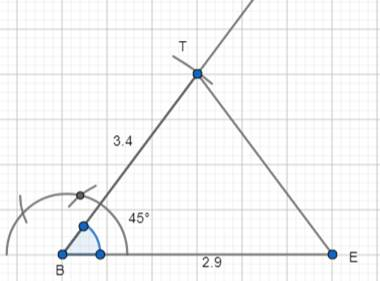Step 3 : To locate the fourth vertex ‘S’ , draw an arc , with center T and radius 2.7cm (TS=2.7cm).

Draw another arc with center E and radius 3.2 cm (ES=3.2cm) which cuts the previous arc at S.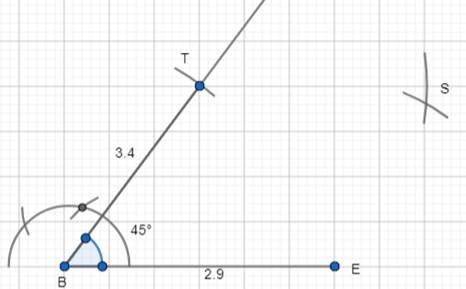Step 4: Join TS and ES to complete the required quadrilateral BEST.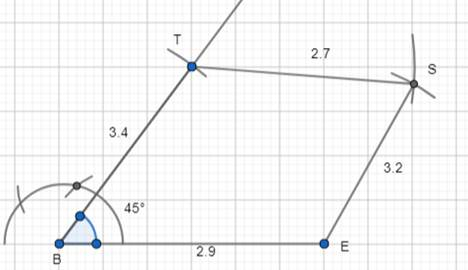Question 3.

Construct the quadrilaterals with the given measurements. And write steps of construction.

Parallelogram PQRS with PQ = 4.5 cm, QR = 3 cm and ∠PQR = 60o.

GIVEN : In Parallelogram PQRS,

PQ = 4.5 cm

QR = 3 cm

∠PQR = 60o

PROCEDURE :

Step 1: Draw a rough sketch of the parallelogram and mark the given measurements.

Here , we are given only 3 measurements. But as PQRS is a parallelogram , we can also write that RS = PQ = 4.5 cm and SP = QR = 3 cm.

(now we got 5 measurements in total)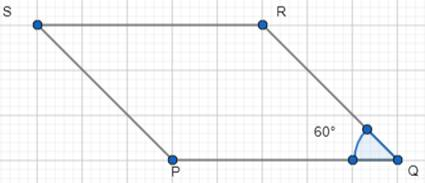Step 2 : Draw ΔPQR using the measures PQ = 4.5 cm , ∠PQR = 60°

and QR = 3 cm.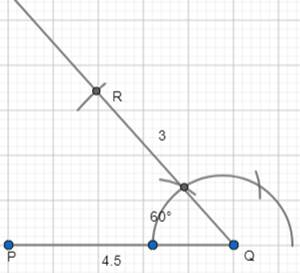Step 3 : Locate the 4th vertex ‘S’ using the other 2 measurements PS = 3 cm and RS = 4.5 cm. To locate the fourth vertex ‘S’ , draw an arc , with center P and radius 3cm (PS=3cm).

Draw another arc with center R and radius 4.5cm (RS=4.5cm) which cuts the previous arc at S.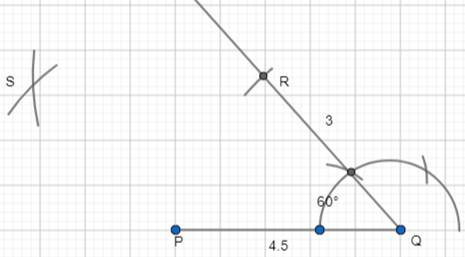Step 4 : Join RS and PS to complete the required parallelogram.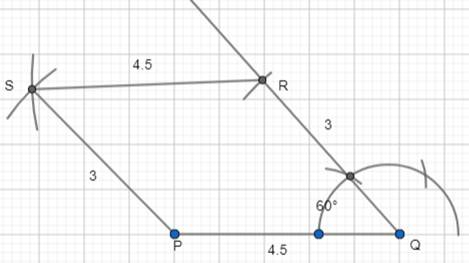Question 4.

Construct the quadrilaterals with the given measurements. And write steps of construction.

Rhombus MATH with AT = 4 cm, ∠MAT = 120o.

GIVEN : In Rhombus MATH,

AT = 4 cm

∠MAT = 120o

PROCEDURE :

Step 1 : Draw a rough sketch of the required quadrilateral and mark the given measurements.

Here , we are given only 2 measurements. But as MATH is a rhombus , we can also write that MA = AT = TH = HM = 4cm.

(now we got 5 measurements in total)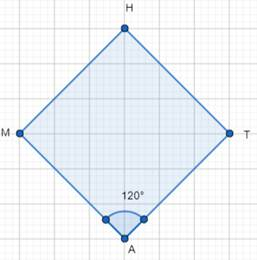Step 2 : Draw ΔMAT using S.A.S property of construction , by taking MA = 4 cm , ∠ MAT = 120° and AT = 4 cm.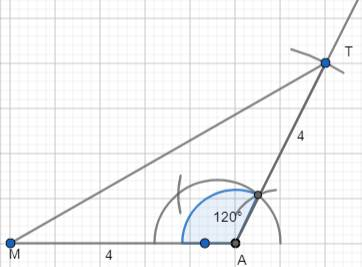Step 3 : To locate the fourth vertex ‘H’ , draw an arc , with center T and radius 4cm (TH=4cm).

Draw another arc with center M and radius 4 cm (MH=4cm) which cuts the previous arc at H.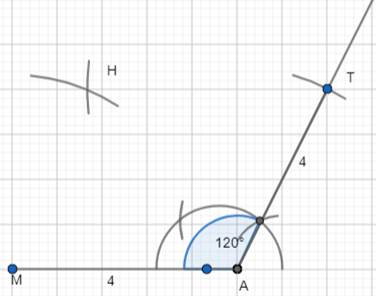Step 4: Join TH and MH to complete the required rhombus MATH.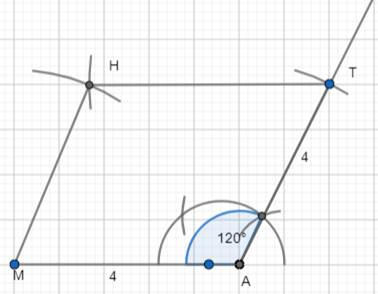Question 5.

Construct the quadrilaterals with the given measurements. And write steps of construction.

Rectangle FLAT with FL = 5 cm, LA = 3 cm.

GIVEN : In Rectangle FLAT,

FL = 5 cm

LA = 3 cm

∠FLA = 90o

PROCEDURE :

Step 1: Draw a rough sketch of the parallelogram and mark the given measurements.

Here , we are given only 3 measurements. But as FLAT is a rectangle , we can also write that FL = AT = 5 cm and LA = TF = 3 cm.

(now we got 5 measurements in total)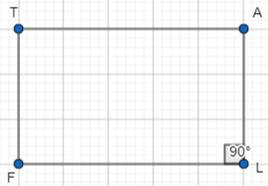Step 2 : Draw ΔFLA using the measures FL = 5 cm , ∠FLA = 90°

and LA = 3 cm.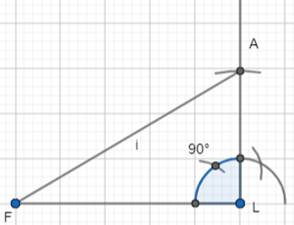Step 3 : Locate the 4th vertex ‘T’ using the other 2 measurements TF = 3 cm and AT = 5 cm. To locate the fourth vertex ‘T’ , draw an arc , with center F and radius 3cm (FT=3cm).

Draw another arc with center A and radius 5cm (AT=5cm) which cuts the previous arc at T.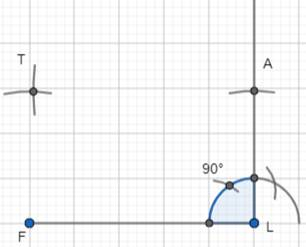Step 4 : Join AT and FT to complete the required rectangle FLAT.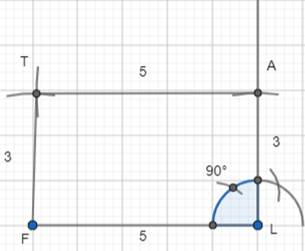Question 6.

Construct the quadrilaterals with the given measurements. And write steps of construction.

Square LUDO with LU = 4.5 cm.

GIVEN : In Square LUDO,

LU = 4.5 cm

∠LUD = 90o

PROCEDURE :

Step 1 : Draw a rough sketch of the required quadrilateral and mark the given measurements.

Here , we are given only 2 measurements. But as LUDO is a square , we can also write that LU = UD = DO = OL = 4.5 cm.

(now we got 5 measurements in total)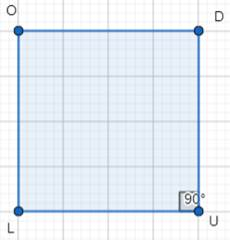Step 2 : Draw ΔLUD using S.A.S property of construction , by taking LU = 4.5 cm , ∠ LUD = 90° and UD = 4.5 cm.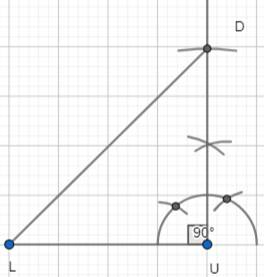Step 3 : To locate the fourth vertex ‘O’ , draw an arc , with center L and radius 4.5cm (LO=4.5cm).

Draw another arc with center D and radius 4.5 cm (DO=4.5cm) which cuts the previous arc at O.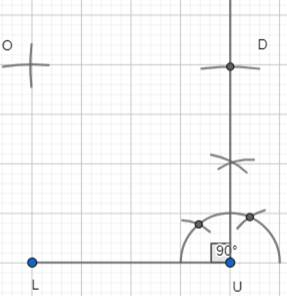Step 4: Join LO and DO to complete the required square LUDO.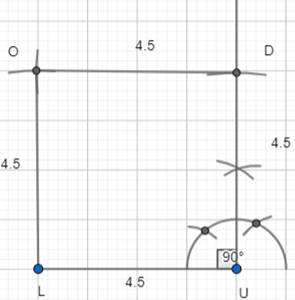###### Exercise 3.2

Question 1.

Construct quadrilateral with the measurements given below:

Quadrilateral ABCD with AB = 4.5 cm, BC = 5.5 cm, CD = 4 cm, AD = 6 cm and AC = 7 cm

GIVEN : In quadrilateral ABCD ,

AB = 4.5 cm

BC = 5.5 cm

CD = 4 cm

AC = 7 cm

PROCEDURE :

Step 1 : draw a rough sketch of the quadrilateral ABCD with the given measurements.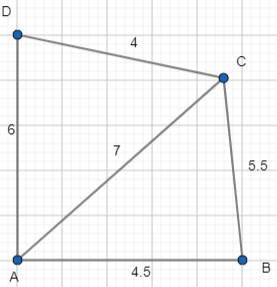Step 2 : Construct ΔABC using SSS construction property with AB = 4.5 cm , BC = 5.5 cm and AC = 7 cm.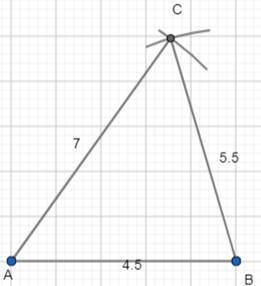Step 3 : we have to locate the 4th vertex ‘D’ . it would be on the other side of AC. So , with center A and radius 6 cm(AD = 6 cm) draw an arc and with center C and radius 4 cm (CD = 4 cm) draw another arc to cut the previous arc at D.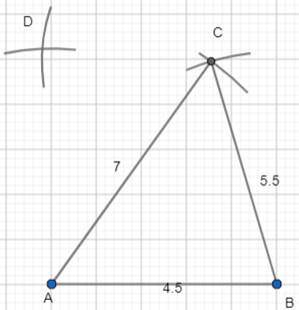Step 4 : join A,D and C,D to complete the quadrilateral ABCD.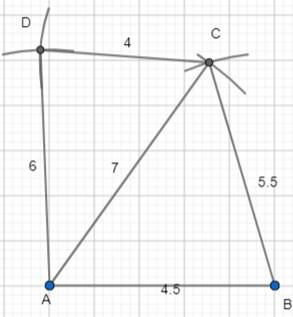Question 2.

Construct quadrilateral with the measurements given below:

Quadrilateral PQRS with PQ = 3.5 cm, QR = 4 cm, RS = 5 cm, PS = 4.5 cm and QS = 6.5 cm

GIVEN : In quadrilateral PQRS ,

PQ = 3.5 cm

QR = 4 cm

RS = 5 cm

PS = 4.5 cm

QS = 6.5 cm

PROCEDURE :

Step 1 : draw a rough sketch of the quadrilateral PQRS with the given measurements.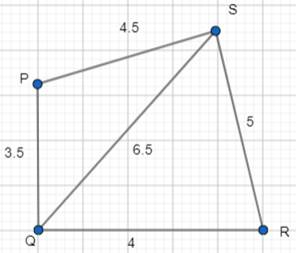Step 2 : Construct ΔPQS using SSS construction property with PQ = 4.5 cm , PS = 4.5 cm and QS = 6.5 cm.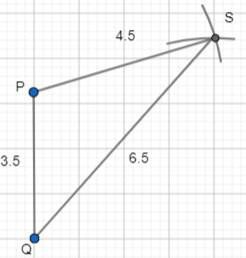Step 3 : we have to locate the 4th vertex ‘R’ . it would be on the other side of QS. So , with center Q and radius 4 cm(QR = 4 cm) draw an arc and with center S and radius 5 cm (SR = 5 cm) draw another arc to cut the previous arc at R.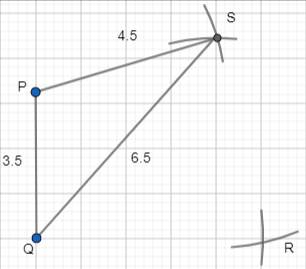Step 4 : join S,R and Q,R to complete the quadrilateral PQRS.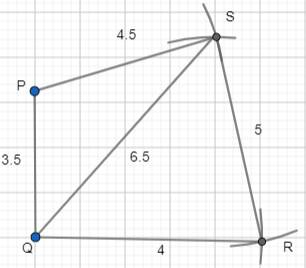Question 3.

Construct quadrilateral with the measurements given below:

Parallelogram ABCD with AB = 6cm, BC = 4.5 cm and BD = 7.5 cm

GIVEN : In Parallelogram ABCD ,

AB = 6 cm

BD = 7.5 cm

BC = 4.5 cm

PROCEDURE :

Step 1 : draw a rough sketch of the Parallelogram ABCD with the given measurements.

Here , we are given only 3 measurements. But as ABCD is a parallelogram , we can also write that AB = CD = 6 cm and BC = AD = 4.5 cm.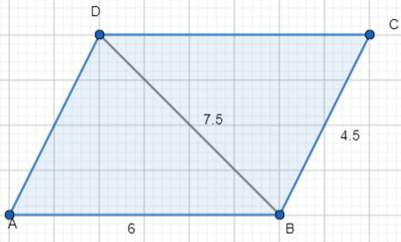Step 2 : Construct ΔABD with AB = 4.5 cm , AD = 4.5 cm and BD = 7.5 cm.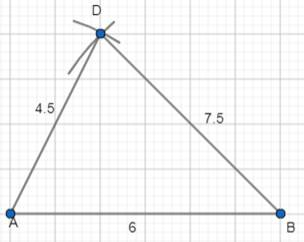Step 3 : we have to locate the 4th vertex ‘C’ . it would be on the other side of BD. So , with center B and radius 4.5 cm(BC = 4.5 cm) draw an arc and with center D and radius 6 cm (CD = 6 cm) draw another arc to cut the previous arc at C.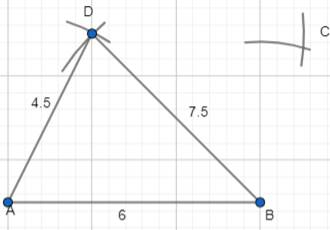Step 4 : join C,B and C,D to complete the quadrilateral ABCD.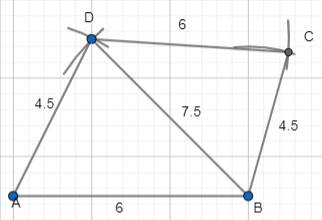Question 4.

Construct quadrilateral with the measurements given below:

Rhombus NICE with NI = 4 cm and IE = 5.6 cm

GIVEN : In Rhombus NICE,

NI = 4 cm

IE = 5.6 cm

PROCEDURE :

Step 1 : draw a rough sketch of the rhombus. Hence all the sides are equal , so , NI = IC = CE = NE = 4 cm and mark the given measurements.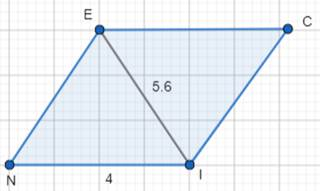Step 2 : draw ΔNIE using SSS construction with measures NI = 4cm , IE= 5.6 cm and EN = 4cm.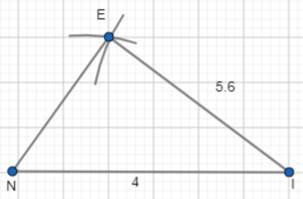Step 3 : we have to locate the 4th vertex ‘C’ . it would be on the other side of IE. So , with center I and radius 4 cm(IC = 4 cm) draw an arc and with center E and radius 4 cm (EC = 4 cm) draw another arc to cut the previous arc at C.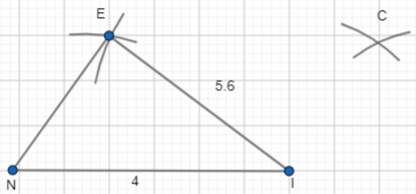Step 4 : Join I,C and C,E to complete the required Rhombus NICE.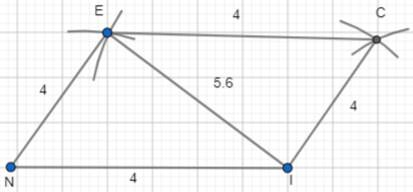###### Exercise 3.3

Question 1.

Construct the quadrilateral with the measurements given below:

Quadrilateral GOLD OL = 7.5 cm, GL = 6 cm, LD = 5 cm, DG = 5.5 cm and OD = 10 cm.

OL = 7.5 cm

GL = 6 cm

LD = 5 cm

DG = 5.5 cm

OD = 10 cm

PROCEDURE :

Step 1 : we first draw the rough sketch of the Quadrilateral GOLD.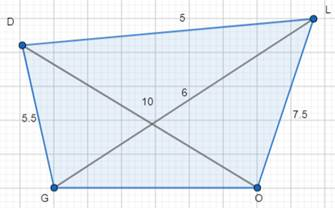Step 2 : draw ΔOLD using SSS construction property with measures OL = 7.5 cm , LD = 5 cm and OD = 10 cm.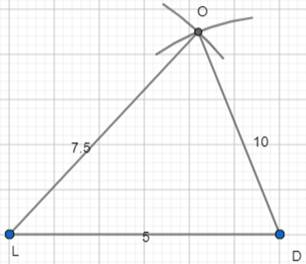Step 3 : with center L and radius 6 cm (LG = 6cm) and with center D and radius 5.5 cm (DG = 5.5 cm) , draw 2 arcs opposite to vertex L to locate G.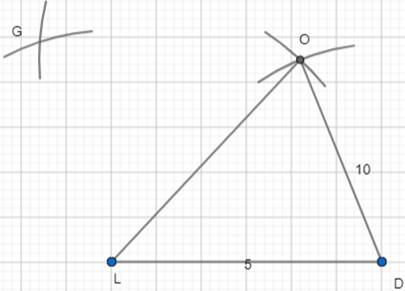Step 4 : Join G,D , L,G and G,O to complete the Quadrilateral GOLD.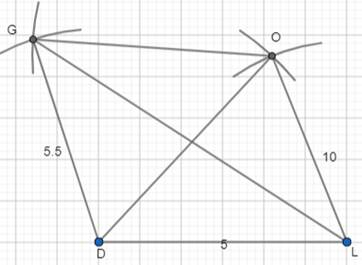Question 2.

Construct the quadrilateral with the measurements given below:

Quadrilateral PQRS, PQ = 4.2 cm, QR = 3 cm, PS = 2.8 cm, PR = 4.5 cm and QS = 5 cm.

PQ = 4.2 cm

QR = 3 cm

PS = 2.8 cm

PR = 4.5 cm

QS = 5 cm

PROCEDURE :

Step 1 : we first draw the rough sketch of the Quadrilateral PQRS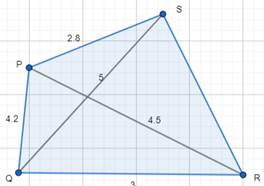Step 2 : draw ΔPQR using SSS construction property with measures PQ = 4.2 cm, QR = 3 cm and PR = 4.5 cm.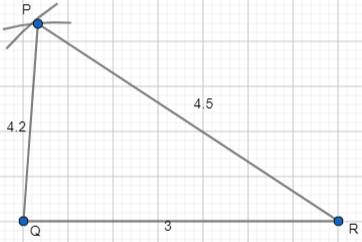Step 3 : with center Q and radius 5 cm (QS = 5 cm) and with center P and radius 2.8 cm (PS = 2.8 cm) , draw 2 arcs opposite to vertex Q to locate S.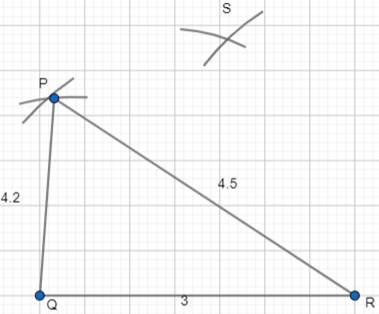Step 4 : Join S,P , Q,S and S,R to complete the Quadrilateral PQRS.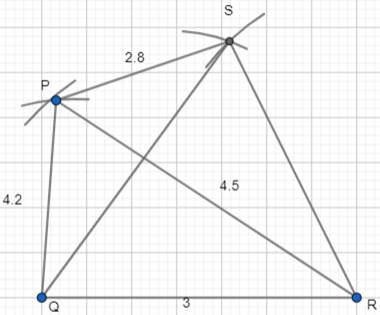###### Exercise 3.4

Question 1.

Construct quadrilaterals with the measurements given below:

Quadrilateral HELP with HE = 6cm, EL = 4.5 cm, ∠H=60o, ∠E =105o and ∠P= 120o.

HE = 6cm

EL = 4.5 cm

∠H=60o

∠E =105o

∠P= 120o

PROCEDURE :

Step 1: draw a rough sketch of the Quadrilateral HELP and mark the given measurements.

As we can see that ∠P is not between the given 2 sides , so we now find the ∠L that is between HE and EL using the property of sum of all angles of a quadrilateral ie. ∠L = 360° - (∠H + ∠E + ∠P) = 360° - (60° + 105° + 120°) = 75°.

∴ ∠L = 75°.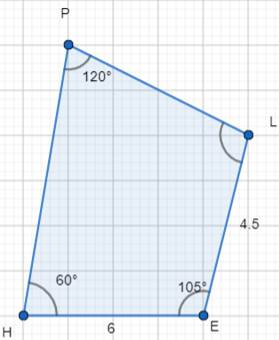Step 2: construct ΔHEL using SAS property of construction model with HE = 6cm , ∠E = 105o and EL = 4.5 cm.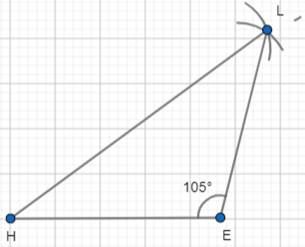Step 3 : Construct ∠H = 60° and draw HY .

how to draw 60° angle ?

an arc is drawn from H . let it intersect HE at H’ . with center H’ and with same radius draw 2 arcs to cut at 2 points A,B which gives 60° and 120° respectively.

So , draw a line from H which passes through A to get the required angle. )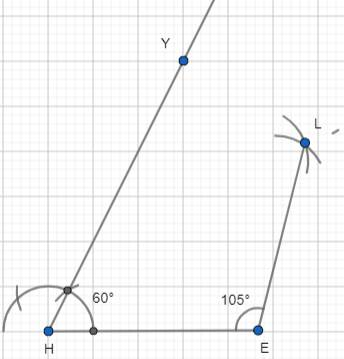Step 4 : construct ∠L = 75o and draw LZ to meet HY at P.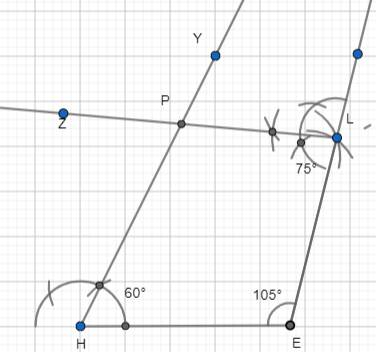Question 2.

Construct quadrilaterals with the measurements given below:

Parallelogram GRAM with GR = AM = 5 cm, RA = MG = 6.2 cm and ∠R = 85o.

GIVEN : In Parallelogram GRAM,

GR = AM = 5 cm

RA = MG = 6.2 cm

∠R = 85o

PROCEDURE :

Step 1 : draw a rough sketch of the parallelogram GRAM and mark the given measurements.

Since the given measurements are not sufficient for construction , we shall find the required measurements using the properties of the parallelogram.

As opposite angles of parallelogram are equal so , ∠R = ∠M = 85o and as the consecutive angles are supplementary so , ∠G = 180° - 85° = 95°.

Thus ∠G = ∠A = 95°.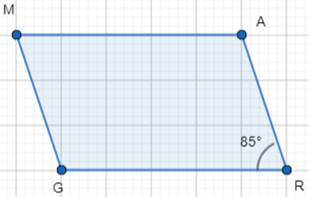Step 2 : construct ΔGRA using SAS property of construction model with GR = 5cm , ∠R = 85o and RA = 6.2 cm.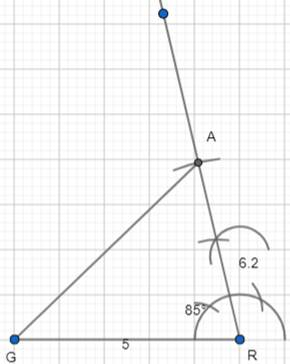Step 3 : construct ∠G = 95° and draw GY || RA.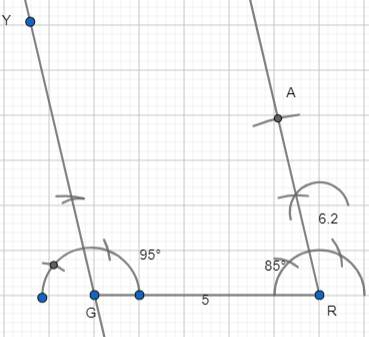Step 4 : construct ∠A = 95° and draw AN to meet GY at M.

GRAM is the required quadrilateral (ie. Parallelogram).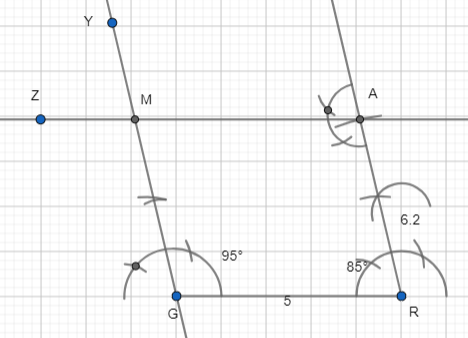Question 3.

Construct quadrilaterals with the measurements given below:

Rectangle FLAG with sides FL = 6cm and LA = 4.2 cm.

GIVEN : In Rectangle FLAG,

FL = 6 cm

LA = 4.2 cm

PROCEDURE :

Step 1 : draw a rough sketch of the Rectangle FLAG and mark the given measurements.

Since the given measurements are not sufficient for construction , we shall find the required measurements using the properties of the RECTANGLE.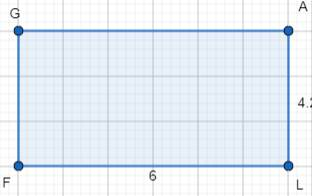As opposite sides of rectangle are equal so, FL = AG = 6cm and LA = GF = 4.2 cm and ∠F = ∠L = ∠A = ∠G = 90°.

Step 2 : construct ΔFLA using SAS property of construction model with FL = 6cm , ∠L = 90o and LA = 4.2 cm.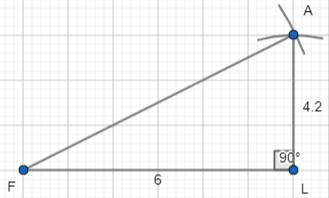Step 3 : construct ∠F = 90° and draw FY || LA.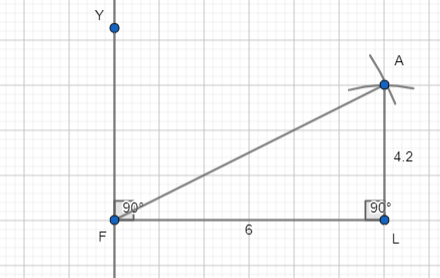Step 4 : construct ∠A = 90° and draw AN to meet FY at G.

FLAG is the required quadrilateral (ie. rectangle).